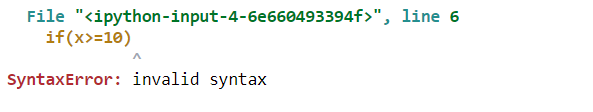top of page

# Python Concepts for Data Science: Errors and ExceptionsErrors are the problems in a program due to which the program will stop the execution. On the other hand, exceptions are raised when some internal events occur which changes the normal flow of the program.

Two types of Error occurs in python

1. Syntax errors

2. Logical errors (Exceptions)

## Syntax errors

When the proper syntax of the language is not followed then syntax error is thrown.

Example

```
# initialize exam score
x = int(input("What is your exam score?"))

# check  whether the student has passed their exam or not
if(x>=10)
print("Congrats! you succeed ")
else print("You did not pass the exam")

```

this code returns a syntax error message because after if statement a colon : is missing.## logical errors(Exception)

Here’s a list of the common exception you’ll come across in Python:

• ZeroDivisionError: raised when you try to divide a number by zero

• IndexError: Raised when the wrong index of a list is retrieved.

• AssertionErrorIt Raised when assert statement fails.

• AttributeError Raised when an attribute assignment is failed.

• KeyError Raised when the key of the dictionary is not found.

• NameError Raised when the variable is not defined.

• TypeError Raised when a function and operation is applied in an incorrect type.

• MemoryError Raised when a program run out of memory.

• ValueError: Raised when the built-in function for a data type has the valid type of arguments, but the arguments have invalid values specified

• Exception: Base class for all exceptions. If you are not sure about which exception may occur, you can use the base class. It will handle all of them.

Example1: ZeroDivisionError

```# initialize the amount variable
marks = 10000

# perform division with 0
a = marks / 0
print(a)```Example2: Index Error

```#initialize a list
a = [1, 2, 3]
print (a) ```Example3: AttributeError

```class Attributes(object):
pass

object = Attributes()
print (object.attribute)```Example4: ImportError

`import module`Example5: KeyError

```array = { 'a':1, 'b':2 }
print (array['c'])```Example6: NameError

```def func():
print (ans)

func()```Example6: TypeError

```arr = ('tuple', ) + 'string'
print (arr)```Example7: ValueError

`print (int('a'))`## Error Handling

When an error and an exception is raised then we handle them with the help of handling methods.

Handling Exceptions with Try/Except/Finally

We can handle error by Try/Except/Finally methods. We write unsafe code in the try, fall back code in except and final code in finally block.

```# put unsafe operation in try block
try:
print("code start")

# unsafe operation perform
print(1 / 0)

# if error occur the it goes in except block
except:
print("an error occurs")

# final code in finally block
finally:
print("GeeksForGeeks")``````try:
a = 10/0
print (a)
except ArithmeticError as e:
print ("This statement is raising an arithmetic exception.")
print(e)
else:
print ("Success.")```Thank you for regarding!

You can find the complete source code here Github